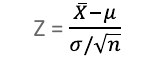Tags
#has-images
Question
How do you find a probability that a variable is greater than a certain value, less than a certain value, or between two values using the central limit theorem?1. Use the formula in image to find Z-value
2. Find areas under the curve for the different Z-values of interest.
3. Add or subtract areas as needed

Tags
#has-images
Question
How do you find a probability that a variable is greater than a certain value, less than a certain value, or between two values using the central limit theorem?
?

Tags
#has-images
Question
How do you find a probability that a variable is greater than a certain value, less than a certain value, or between two values using the central limit theorem?1. Use the formula in image to find Z-value
2. Find areas under the curve for the different Z-values of interest.
3. Add or subtract areas as needed
If you want to change selection, open document below and click on "Move attachment"

#### pdf

owner: naxplast06 - (no access) - Deviant S. - The Practically Cheating Statistics Handbook (2010, CreateSpace Independent Publishing Platform).pdf, p105

#### Summary

status measured difficulty not learned 37% [default] 0

No repetitions